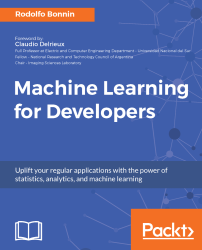•#### Machine Learning for Developers#### Overview of this book

Most of us have heard about the term Machine Learning, but surprisingly the question frequently asked by developers across the globe is, “How do I get started in Machine Learning?”. One reason could be attributed to the vastness of the subject area because people often get overwhelmed by the abstractness of ML and terms such as regression, supervised learning, probability density function, and so on. This book is a systematic guide teaching you how to implement various Machine Learning techniques and their day-to-day application and development. You will start with the very basics of data and mathematical models in easy-to-follow language that you are familiar with; you will feel at home while implementing the examples. The book will introduce you to various libraries and frameworks used in the world of Machine Learning, and then, without wasting any time, you will get to the point and implement Regression, Clustering, classification, Neural networks, and more with fun examples. As you get to grips with the techniques, you’ll learn to implement those concepts to solve real-world scenarios for ML applications such as image analysis, Natural Language processing, and anomaly detections of time series data. By the end of the book, you will have learned various ML techniques to develop more efficient and intelligent applications.
PrefaceFree Chapter
Introduction - Machine Learning and Statistical ScienceThe Learning ProcessClusteringLinear and Logistic RegressionNeural NetworksConvolutional Neural NetworksRecurrent Neural NetworksRecent Models and DevelopmentsSoftware Installation and Configuration# Loss function definition

This machine learning process step is also very important because it provides a distinctive measure of the quality of your model, and if wrongly chosen, it could either ruin the accuracy of the model or its efficiency in the speed of convergence.

Expressed in a simple way, the loss function is a function that measures the distance from the model's estimated value to the real expected value.

An important fact that we have to take into account is that the objective of almost all of the models is to minimize the error function, and for this, we need it to be differentiable, and the derivative of the error function should be as simple as possible.

Another fact is that when the model gets increasingly complex, the derivative of the error will also get more complex, so we will need to approximate solutions for the derivatives with iterative methods...